Open in App
Not now

# Histograms and Density Plots in Python

• Difficulty Level : Easy
• Last Updated : 31 Jul, 2021

Prerequisites: Seaborn

The histogram is the graphical representation that organizes a group of data points into the specified range. Creating the histogram provides the Visual representation of data distribution. By using a histogram we can represent a large amount of data, and its frequency.

Density Plot is the continuous and smoothed version of the Histogram estimated from the data. It is estimated through Kernel Density Estimation.

In this method Kernel (continuous curve) is drawn at every individual data point and then all these curves are added together to make a single smoothened density estimation. Histogram fails when we want to compare the data distribution of a single variable over the multiple categories at that time Density Plot is useful for visualizing the data.

### Approach:

• Import the necessary libraries.
• Create or import a dataset from seaborn library.
• Select the column for which we have to make a plot.
• For making the plot we are using distplot() function provided by seaborn library for plotting Histogram and Density Plot together in which we have to pass the dataset column.
• We can also make Histogram and Density Plot individually using distplot() function according to our needs.
• For creating Histogram individually we have to pass hist=False as a parameter in the distplot() function.
• For creating Density Plot individually we have to pass kde=False as a parameter in the distplot() function.
• Now after making the plot we have to visualize that, so for visualization, we have to use show() function provided by matplotlib.pyplot library.

For plotting the Histogram and Density Plots together we are using diamond and iris dataset provided by seaborn library.

Example 1: Importing the dataset and Print them.

## Python

 `# importing seaborn library``import` `seaborn as sns` `# importing dataset from the library``df ``=` `sns.load_dataset(``'diamonds'``)` `# printing the dataset``df`

Output: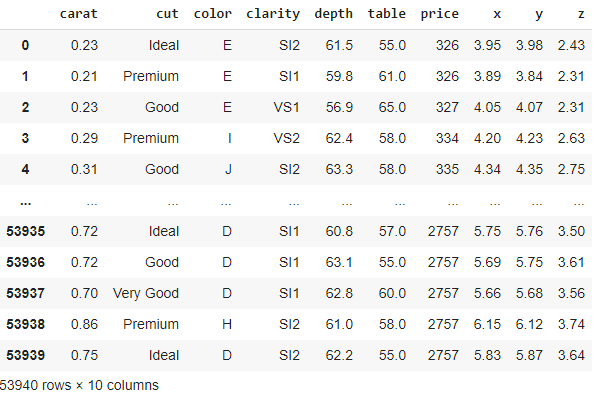Example 2: Plotting the Histogram using seaborn library on the default setting.

## Python

 `# importing necessary libraries``import` `seaborn as sns``import` `matplotlib.pyplot as plt` `# importing diamond dataset from the library``df ``=` `sns.load_dataset(``'diamonds'``)` `# plotting histogram for carat using distplot()``sns.distplot(a``=``df.carat, kde``=``False``)` `# visualizing plot using matplotlib.pyplot library``plt.show()`

Output: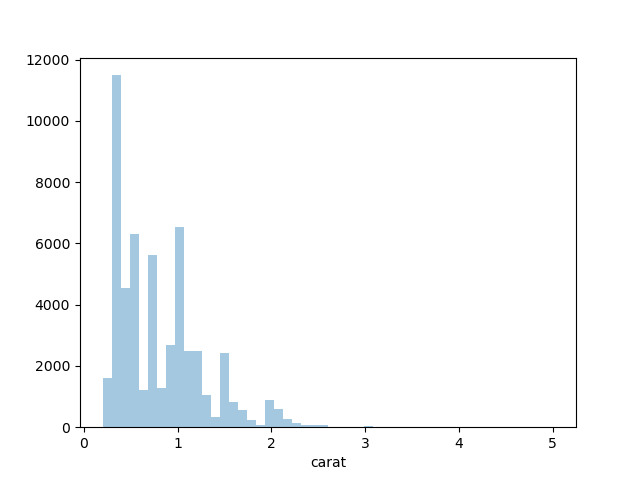Example 3: Plotting the Density using seaborn library on the default setting.

## Python

 `# importing libraries``import` `seaborn as sns``import` `matplotlib.pyplot as plt` `# importing diamond dataset from the library``df ``=` `sns.load_dataset(``'diamonds'``)` `# plotting density plot for carat using distplot()``sns.distplot(a``=``df.carat, hist``=``False``)` `# visualizing plot using matplotlib.pyplot library``plt.show()`

Output: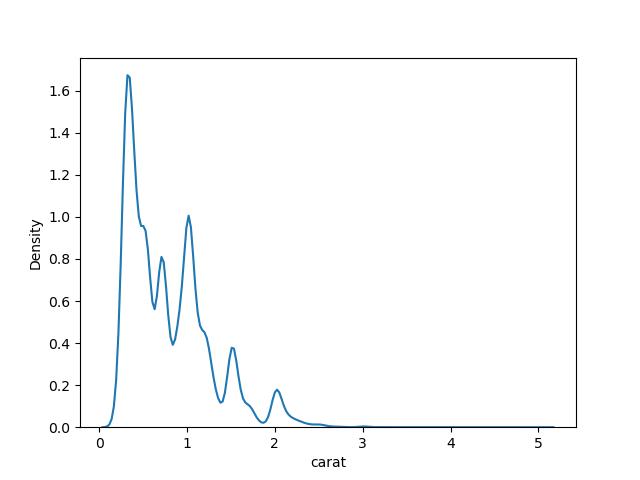Example 4: Plotting Histogram and Density Plot together on default settings.

## Python

 `# importing libraries``import` `seaborn as sns``import` `matplotlib.pyplot as plt` `# importing diamond dataset from the library``df ``=` `sns.load_dataset(``'diamonds'``)` `# plotting histogram and density``# plot for carat using distplot()``sns.distplot(a``=``df.carat)` `# visualizing plot using matplotlib.pyplot library``plt.show()`

Output: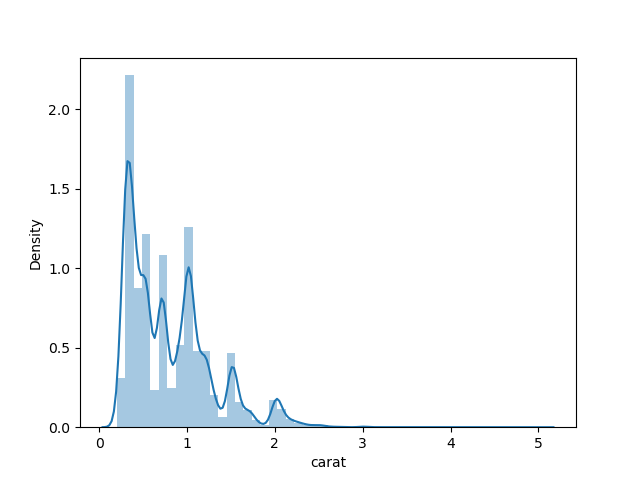Example 5: Plotting Histogram and Density Plot together by setting bins and color.

## Python

 `# importing libraries``import` `seaborn as sns``import` `matplotlib.pyplot as plt` `# importing diamond dataset from the library``df ``=` `sns.load_dataset(``'diamonds'``)` `# plotting histogram and density plot``# for carat using distplot() by setting color``sns.distplot(a``=``df.carat, bins``=``40``, color``=``'purple'``,``             ``hist_kws``=``{``"edgecolor"``: ``'black'``})` `# visualizing plot using matplotlib.pyplot library``plt.show()`

Output: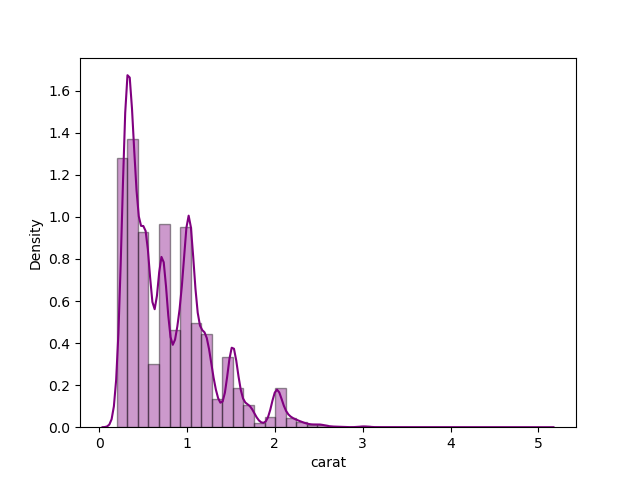Example 6: Plotting Histogram and Density Plot together using Iris dataset.

## Python

 `# importing libraries``import` `seaborn as sns``import` `matplotlib.pyplot as plt` `# importing iris dataset from the library``df2 ``=` `sns.load_dataset(``'iris'``)` `# plotting histogram and density plot for``# petal length using distplot() by setting color``sns.distplot(a``=``df2.petal_length, color``=``'green'``,``             ``hist_kws``=``{``"edgecolor"``: ``'black'``})` `# visualizing plot using matplotlib.pyplot library``plt.show()`

Output: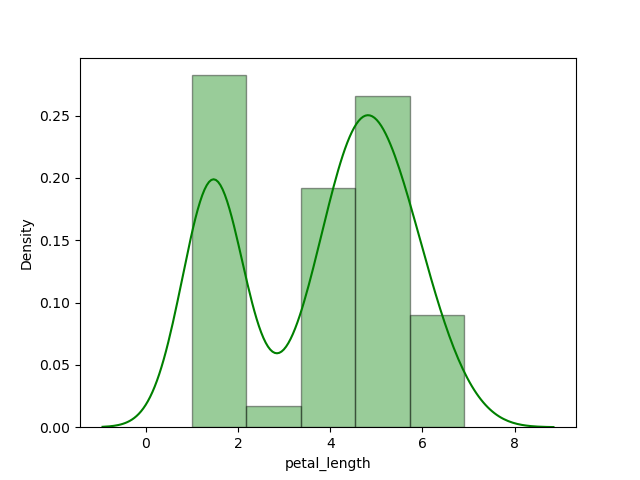We can also print the iris dataset by adding one line of code i.e, print(df2), and dataset looks like.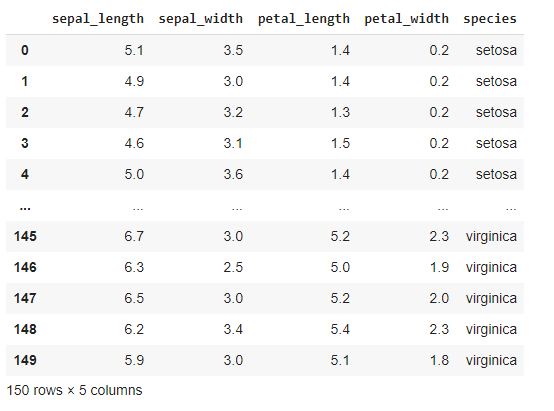Example 7: Plotting Histogram and Density Plot together on sepal length.

## Python

 `# importing libraries``import` `seaborn as sns``import` `matplotlib.pyplot as plt` `# importing iris dataset from the library``df2 ``=` `sns.load_dataset(``'iris'``)` `# plotting histogram and density plot for``# sepal width using distplot() by setting color``sns.distplot(a``=``df2.sepal_width, color``=``'red'``,``             ``hist_kws``=``{``"edgecolor"``: ``'white'``})` `# visualizing plot using matplotlib.pyplot library``plt.show()`

Output: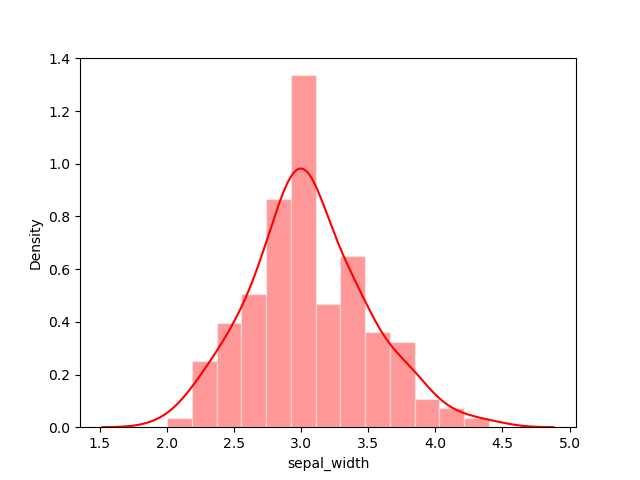In this way, we can plot Histogram and Density Plot together on any dataset columns according to our needs.

My Personal Notes arrow_drop_up